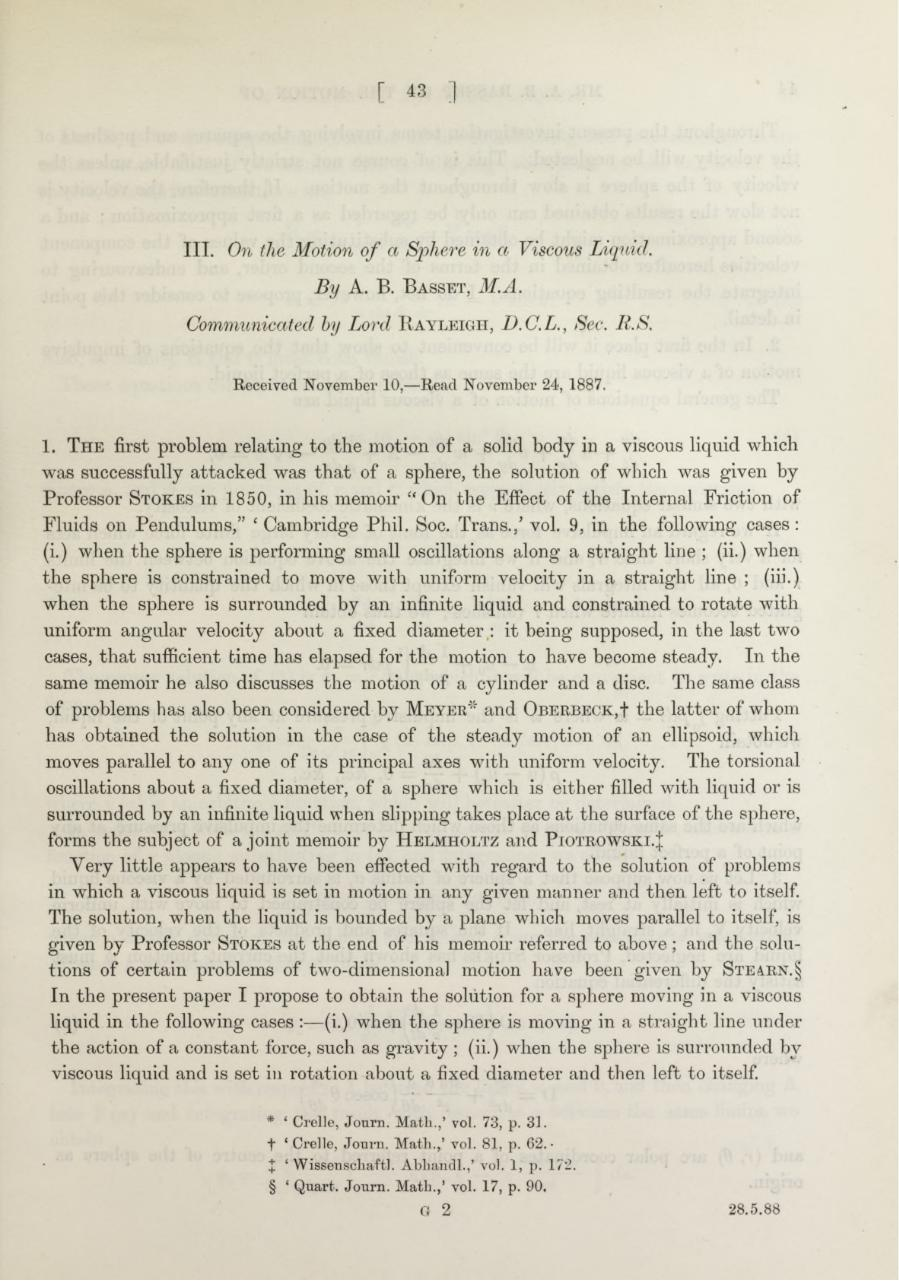# PDF Archive

Easily share your PDF documents with your contacts, on the Web and Social Networks.

## A. B. Basset. On the motion of a sphere in a viscous liquid.pdfPage 12321

#### Text preview

III. On

theMotion o f a Sphere in a Viscous
B y A. B. B a sset ,

Communicated by Lord R a y l e ig h , D.C.L., Sec,

1. T h e first problem relating to the motion of a solid body in a viscous liquid which
was successfully attacked was th a t of a sphere, the solution of which was given by
Professor S to k es in 1850, in his memoir “ On the Effect of the Internal Friction of
Fluids on Pendulum s,” ‘ Cambridge Phil. Soc. T rans./ vol. 9, in the following cases:
(i.) when the sphere is performing small oscillations along a straig h t line ; (ii.) when
th e sphere is constrained to move w ith uniform velocity in a straig h t line ; (iii.)
when th e sphere is surrounded by an infinite liquid and constrained to rotate with
uniform angular velocity about a fixed diam eter : it being supposed, in the last two
cases, th a t sufficient time has elapsed for the motion to have become steady. In the
same memoir he also discusses the motion of a cylinder and a disc. The same class
of problems has also been considered by M e y e r * and O b e r b e c k ,! the latter of whom
has obtained th e solution in the case of the steady motion of an ellipsoid, which
moves parallel to any one of its principal axes with uniform velocity. The torsional
oscillations about a fixed diameter, of a sphere which is either filled w ith liquid or is
surrounded by an infinite liquid when slipping takes place a t the surface of the sphere,
forms th e subject of a joint memoir by H elm holtz and P io t r o w s k i . |
Very little appears to have been effected with regard to the solution of problems
in which a viscous liquid is set in motion in any given m anner and then left to itself.
The solution, when the liquid is bounded by a plane which moves parallel to itself, is
given by Professor S tokes a t the end of his memoir referred to above; and the solu­
tions of certain problems of two-dimensional motion have been given by S t e a r n .§
In th e present paper I propose to obtain the solution for a sphere moving in a viscous
liquid in the following cases :— (i.) when the sphere is moving in a straight line under
th e action of a constant force, such as gravity ; (ii.) when the sphere is surrounded by
viscous liquid and is set in rotation about a fixed diam eter and then left to itself.*§
*
t
J
§

‘ Crelle, Journ. M ath.,’ vol. 73, p. 31.
‘ Crelle, Joui'ii. M ath.,’ vol. 81, p. 62.*
‘ Wissenscliaftl. Abhandl.,’ vol. 1, p. 172.
‘ Quart. Journ. M ath.,’ vol. 17, p. 90.
G

2

28.5.88